Related Articles

# Class 10 NCERT Solutions- Chapter 12 Areas Related to Circles – Exercise 12.3 | Set 2

• Last Updated : 05 Apr, 2021

### Question 11. On a square handkerchief, nine circular designs each of radius 7 cm are made (see Fig.). Find the area of the remaining portion of the handkerchief.

Solution:

=6*7

=42 cm

Area of shaded region=Area of square – Area of 9 circles

=side*side – 9πr2

=42*42 – 9*22/7*7*7

=1764-1386

=378 cm2

The area of the remaining portion of the handkerchief =378 cm2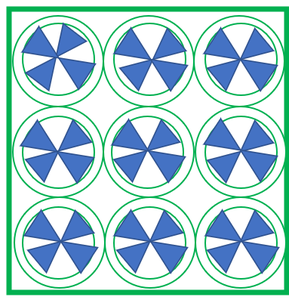### Question 12. In Fig., OACB is a quadrant of a circle with Centre O and radius 3.5 cm. If OD = 2 cm, find the area of the

Solution:

=1/4πr2

=1/4*22/7*3.5*3.5

=38.5/4

=9.625 cm2

=9.625-1/2*BO*OD

=9.625-1/2*3.5*2

=9.625-3.5

=6.125 cm2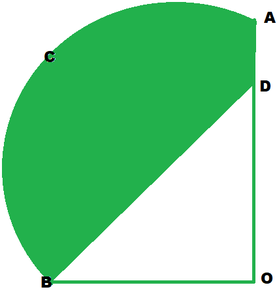### Question 13. In Fig., a square OABC is inscribed in a quadrant OPBQ. If OA = 20 cm, find the area of the shaded region. (Use π = 3.14)

Solution:

By Pythagoras theorem,

OB2=DA2+AB2

BO2=(20)2+(20)2

OB2=400+400

OB2=800

OB=√800

OB=√(2*2*2*2*5*5)

OB=2*2*5√2

OB=20√2

=1/4πr2 – side*side

=1/4*3.14*20√2*20√2-20*20

=1/4*3.14*800-400

=1*3.14

=22cm2

=1/4*3.14

The area of shaded region is =1/4*3.14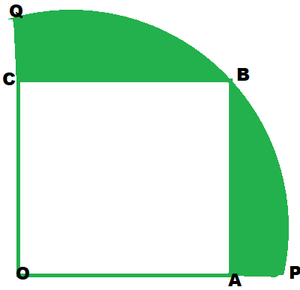### Question 14. AB and CD are respectively arcs of two concentric circles of radii 21 cm and 7 cm and centre O (see Fig.). If ∠AOB = 30°, find the area of the shaded region.

Solution:

Area of shaded region=Area of sector AOB-Area of sector COD

=θ/(360°) πR2-θ/(360°) πr2

=θ/(360°) π[R2-r2]

=30°/360*22/7[(21)2-(7)2]

=1/12*22/7*28*14

=308/3cm2

=102.66cm2

The area of shaded region 102.66cm2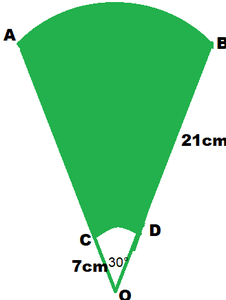### Question 15. In Fig., ABC is a quadrant of a circle of radius 14 cm and a semicircle is drawn with BC as diameter. Find the area of the shaded region.

Solution:

Area of segment=Area of quadrant -Area of ∆BAC

=1/4πr2-1/2*AC*AB

=1/4*22/7*14*14-1/2*14*14

=11*14-98

=154-98

=56 cm2

Semicircle R=?

In rt. ∆BAC, By Pythagoras theorem,

BC2=AB2+BC2

BC2= (14)2+(14)2

BC=√((14)2+(14)2)

BC=√((14)2[1+1] )

BC=14√2

∴Diameter of semicircle=14√2cm

Area of semicircle =1/2πR2

=1/2*22/7*7√2*7√2

=22*7

=154 cm2

Area of shaded region=Area of semicircle-Area of segment

=154-56 cm2

=98 cm2

The area of shaded region is =98cm2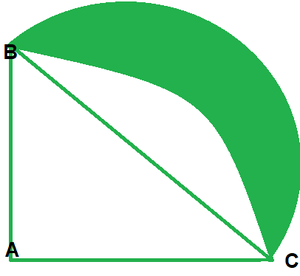### Question 16. Calculate the area of the designed region in Fig. common between the two quadrants of circles of radius 8 cm each.

Solution:

Area of design=Area of 2 quadrant -Area of square

=2*1/4πr2-side*side

=1/2*22/7*8*8-8*8

=704/7-64

=100.57-64

=36.57cm2

Area designed region in figure is 36.57cm2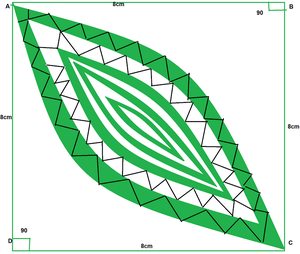Attention reader! Don’t stop learning now. Join the First-Step-to-DSA Course for Class 9 to 12 students , specifically designed to introduce data structures and algorithms to the class 9 to 12 students

My Personal Notes arrow_drop_up